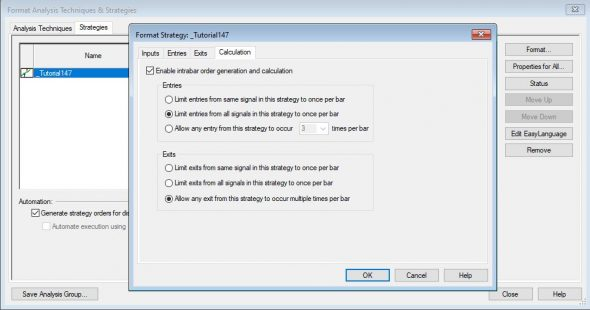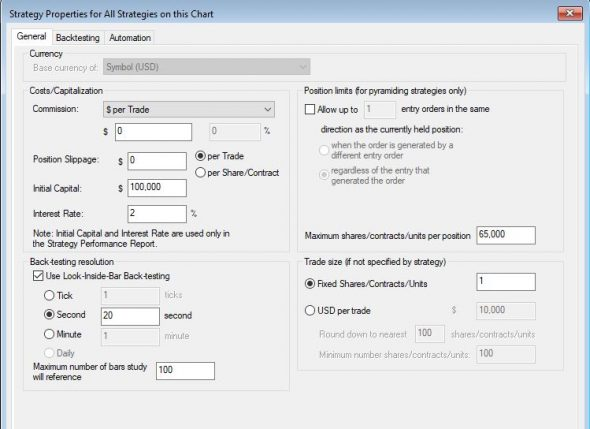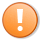# Tutorial 147 | Look-inside-bar-back-testing and intra bar order generation and calculation

Tradestation users often wonder why the performance of a strategy is one thing, but when adjusting the Look-inside-bar-back-test and Intra bar order generation and calculation the performance can be radically different. Tutorial 147 seeks to demonstrate the differences to strategy bar data when Look-inside-bar-back-test and Intra bar order generation and calculation are selected. To do so the tutorial uses a very simple moving average cross-over strategy. The demonstration program and workspace (for TradeStation 9.5 and 10) may be downloaded by Gold Pass members at no cost (see below).

The following short video shows some of the differences:

### Video demonstration of tutorial 147

Tutorial 147 uses the following print statement to show the moving average values, whether crossovers have occurred and the market position for each tick:

```//If GetAppInfo( aiRealTimeCalc ) = 1 and BarInterval = 1 then // Real time bars and 1 minute bar
//If GetAppInfo( aiRealTimeCalc ) = 0 and BarInterval = 1 then // Historic bars and 1 minute bar

//If GetAppInfo( aiRealTimeCalc ) = 1 and BarInterval = 2 then // Real time bars and 2 minute bar
//If GetAppInfo( aiRealTimeCalc ) = 0 and BarInterval = 2 then // Historic bars and 2 minute bar

If GetAppInfo( aiRealTimeCalc ) = 1 and BarInterval = 3 then // Real time bars and 3 minute bar
//If GetAppInfo( aiRealTimeCalc ) = 0 and BarInterval = 3 then // Historic bars and 3 minute bar

Print( Barinterval," ",D, T, " MovAv1 ", MovAv1," MovAv2 ", MovAv2," MovAv1 crosses above MovAv2 ",MovAv1 crosses above MovAv2,
" MovAv1 crosses below MovAv2 ",MovAv1 crosses below MovAv2, " MP ", MarketPosition( 0 ) );
```

In this tutorial the options are selected using the following dialogs:Enable intra bar order generation and calculation is selected by clicking Format-Strategies, then clicking the Format button, and then selecting the Calculation tabLook-inside-bar-backtesting is selected by clicking Format-Strategies, then the Properties for all button. The setting is towards the bottom of the dialog, on the left.
The three combinations looked at are:
1. Look-inside-bar-backtesting OFF, Enable intra bar order generation and calculation OFF Look-inside-bar-backtesting OFF,
2. Enable intra bar order generation and calculation ON, Look-inside-bar-backtesting ON,
3. Enable intra bar order generation and calculation ON

### Inputs

```double Price( C ), // Price used in moving average calculation int Len1( 5 ), // Length of fast moving average int Len2( 14 ); // Length of slow moving average```This content is for members only.